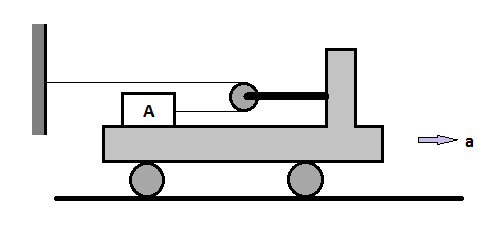# Lets drive to physicsIf flat car is given an acceleration $\text{a} = 3\text{ ms}^{-2}$ starting from rest, compute tension (in $N$) in the light inextensible string connected to block $A$ of mass $30\text{ kg}$. Coefficient of friction between block and flat car is $\mu = 0.50$.

Neglect mass of pulley and its friction. Take $g = 10\text{ ms}^{-2}$.

×

Problem Loading...

Note Loading...

Set Loading...